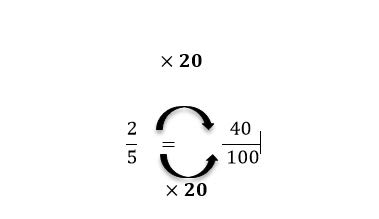## Step 1: Determine the closest factor you can multiply to the denominator to end up with a power of 10

The first thing you must do is express the fraction as the quotient when we divide the numerator by the denominator.

Given a/b : find a factor to multiply to b so you end up with 10, 100, 1000 or any powers of 10.

## Step 2: Multiply that factor to both numerator and denominator

Multiply factor to both a and b.

## Step 3: Count the number of zeroes in the denominator

Write down the numerator as a whole number and move the same number of places from the right. Count zeroes in b, then move the same number of places to 0.

## Examples of how to convert fractions to decimals

Q1) What is the value of 2/5 in decimal?

5×20=100

Multiply 100 to both numerator and denominator100 has two zeroes so move two decimal places to the right of 40. 2/5=0.40